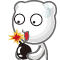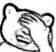# 博客

## UOJ NOI Round #2 题解

2017-07-13 13:39:31 By C_SUNSHINE

MysteryMan 是谁呢？

## Day1

### UOJ拯救计划

from MysteryMan， 题解 by ydc

### 排兵布阵

from MysteryMan， 题解 by jiry_2

C_SUNSHINE：“行吧我石乐志……”

#### 算法三

##### 关于去重

（不过 UNR 传统是 T2 最难？）

C_SUNSHINE：“Exactly!”

### 黎明前的巧克力

from sevenkplus， 题解 by C_SUNSHINE

#### 算法三

$f_{i-1,j} \rightarrow f_{i,j}$

$f_{i-1,j}\times 2 \rightarrow f_{i,j~xor~a_i}$

#### 算法六

$$FWT(F)(n) = \sum_{k=0}^{2^m-1} \left(F(k) \prod_{j=0}^{m-1}(-1)^{n_jk_j}\right)$$

$$FWT(F)(n) = \sum_{k=0}^{2^m-1} F(k) (-1)^{bitcount(n_j~and~k_j)}$$

#### 算法八

$$FWT(F)(n) = \sum_{k=0}^{2^m-1} \left(F(k) \prod_{j=0}^{m-1}(-1)^{n_jk_j}\right)$$

## Day2

### 积劳成疾

from MysteryMan， 题解 by C_SUNSHINE

### 梦中的题面

La tâche est écrite par ftiasch,

et la solution est écrite par matthew99

### 解开尘封的仪式

from sqc， 题解 by sqc

sqc太菜了，他的提答被A穿了，就当送分好了……#### 测试点8

（泥萌怎么都会这个点啊#### 测试点9

（为了减小数据包的大小，删了一些点，这个点出锅了，被乱搞搞过去了啊## 评论

C_SUNSHINE

matthew99

l__ZeRo_t

yanQval

orzlxl

hanyuu

ddddddpppppp

lethalboy

liu_cheng_ao

_Itachi

tayloronline

liu_runda

xumingkuan
T1出题人真良心啊，给了我们这些忘判m=0直接如果k模3不余2就输出0的可怜选手10分，有效防止了爆零，非常贴合NOI d1t1难度
zzyu5ds

WAAutoMaton

g19zwk

xuhaike

AntiLeaf

150137

wangxiuhan